# The BriefA Blog about the LSAT, Law School and Beyond

Circle graphs (also called pie charts) let you see the relative amounts of different categories. For example, if you run a local grocery store and want to see where your sales are coming from (perhaps because you are considering whether to re-allocate floor space), you might look at a chart like the following: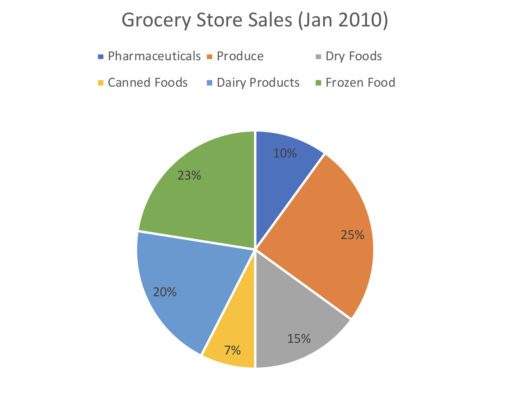and conclude that as most of your sales come from produce, you may want to allocate more space to new kinds of produce.

Now, when reading a circle graph, the percentages of the different sections will generally be labelled as in the above. So circle graph tells you that in January of 2010, 23% of all sales were from frozen foods, 10% of all sales were from pharmaceuticals, and so on.

Now, if you are given the actual value (as opposed to the proportion) of any category, you can find the value for each category. So, for example:

Example 1

Suppose that in January of 2010, the grocery store sold $23,000 worth of frozen foods. How many dollars worth of canned foods did they sell? We can also use circle graphs to determine various trends. For example, by comparing the following charts: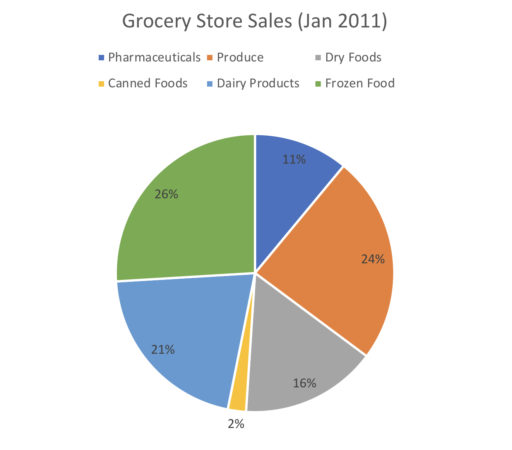we can conclude that the proportion of revenue from canned foods drastically shrunk from January 2010 to 2011. Example 2 From January 2010 to January 2011, which category grew the most as a proportion of total sales? Now, if we know the actual value of some category in 2010 and the actual value of some category in 2011, we can calculate the value of each category and 2010 and 2011. Thus, we can calculate the absolute increase in revenue for any particular category as follows: Example 3 Suppose in January 2010, the total amount of goods sold was$200,000. In January 2011, the amount of canned foods sold was $6,000. Find the absolute change from 2010 to 2011 in the amount of dairy products sold. Practice Problems 1. Suppose produce sales were, in absolute terms,$10,000 greater than pharmaceutical sales in January 2010. What was the total amount of sales for all goods?2. Overall sales in January 2010 were \$100,000. How much more revenue was generated by dry foods as compared to canned foods?3. Suppose dry foods in January 2010 were twice as large, in absolute terms, as pharmaceutical sales in January 2011. What is the ratio of overall sales from January 2010 to January 2011?Comment on this

A box plot is so named for its iconic shape: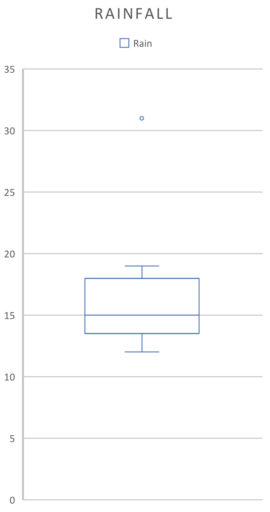(They can also be laid out horizontally). The parts of the graph correspond to: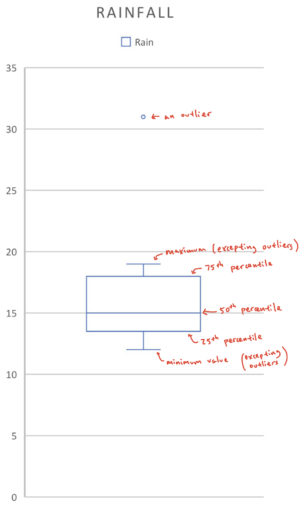Looking at a box plot can give you a quick sense of what the distribution of the data looks like. For example:

Example 1

Find the 25th percentile, 50th percentile, 75th percentile, range, and median for the below boxplot: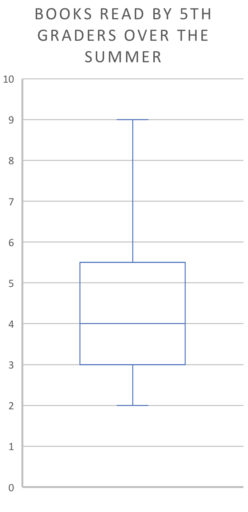Example 2

The following chart represents the books read by the 500 fifth-graders in a school over the summer. Suppose no student read exactly 4 books. How many students read more than 4 books?Example 3

The following chart represents the books read by the 500 fifth-graders in a school over the summer. What is the approximate number of students that have read between 3 and 4 books?Practice Problems

1. The following chart represents the books read by the 100 fifth-graders in a school over the summer. What is the approximate number of students that have read more than 5 books? (We assume that people can only read positive integers of books).2. The following chart represents the books read by the 500 fifth-graders in a school over the summer. Suppose 7 is the 80th percentile of this data. Approximately how many people read between 6 and 7 books? (We assume that people can only read positive integers of books).3. Researchers measured, over 36 months, how often it rained in a month. About how many months had rain from between 13 and 18 days?